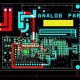# What is Zener Diode & Its Application?

In this tutorial, we are going to learn about zener diode & its application.

Zener Diode – A zener diode is a special type of P-N junction diode and it can also work in reverse bias that makes it special.

In reverse bias zener diode works as a voltage regulator.

V-I characteristics of Zener Diode:

A voltage regulator circuit keeps the load voltage constant irrespective changes in input voltage or load resistance.

Zener Diode Application:

Zener Diode as a Voltage Regulator:

Voltage Regulator –

• It is combination of elements designed to ensure that the output voltage remains constant.
• The resistor limits the total current flowing to the diode and the load. The load is connected across the diode.

Vo  = VZ

VS  – VR – VZ = 0

VR = VS – VZ

IR  = VS – VZ / RS

Kirchoff’s law :

IR  = IZ + IL   , IZ = IR – IL

Ideal Approximation of Zener diode:

Zener diode operating in breakdown region ideally act like a battery of value equal to breakdown voltage.

Second Approximation of Zener diode:

Total Voltage drop across diode is equal to the breakdown voltage and voltage drop across zener resistance (RZ).

Here below are the circuit for zener diode working

Regulating action of zener diode with varying input voltage:

When VI increasing: Vo  = VZ as long as

When VI decreasing: Vo  = VZ as long as

IZ  = I – IL

Regulating action of zener diode with varying load resistance:

1. When RL increases , If RL increases then IL decrease But I remains constant.

I  = IZ + IL

Since IL decrease then IZ increases

Therefore output voltage Vo = VZ as long as IZ < IZMAX

• When RL decreases, If RL decreases then IL increase But I remains constant.

Since IL increase then IZ decreases

Vo  = VZ as long as